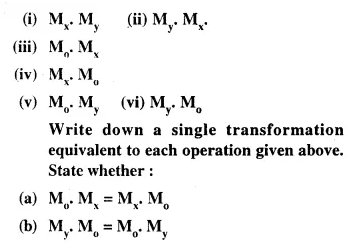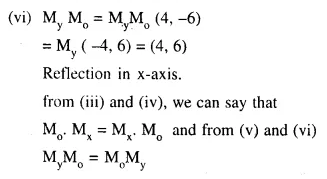## Selina Concise Mathematics Class 10 ICSE Solutions Chapter 12 Reflection (In x-axis, y-axis, x=a, y=a and the origin ; Invariant Points) Ex 12A

These Solutions are part of Selina Concise Mathematics Class 10 ICSE Solutions. Here we have given Selina Concise Mathematics Class 10 ICSE Solutions Chapter 12 Reflection Ex 12A.

Other Exercises

Question 1.
Complete the following table.Solution:
We can complete the blanks as under
(a) Reflection in origin
(b) (4, -2)
(c) (0, 6)
(d) Reflection in origin
(e) Reflection in y-axis.

Question 2.
A point P is its own image under the reflection in a line l. Describe the position of the point P with respect to the line l.
Solution:The image of the point is itself the point P with respect to a line l, Then the point lies in the line l.

Question 3.
State the co-ordinates of the following points under reflection in x-axis:
(i) (3, 2)
(ii) (-5, 4)
(iii) (0, 0)
Solution:
The co-ordinates of the given points under reflection in x-axis are as under :
(i) (3, -2)
(ii) (-5, -4)
(iii) (0, 0).

Question 4.
State the co-ordinates of the following points under reflection in y-axis :
(i) (6, -3)
(ii) (-1, 0)
(iii) (-8, -2)
Solution:
The co-ordinates of the points under reflection in y-axis are :
(i) (-6, -3)
(ii) (1, 0)
(iii) (8, -2)

Question 5.
State the co-ordinates of the following points under reflection in origin :
(i) (-2, -4)
(ii) (- 2, 7)
(iii) (0, 0)
Solution:
The co-ordinates of the points under reflection in origin are :
(i) (2, 4)
(ii) (2, -7)
(iii) (0, 0)

Question 6.
State the co-ordinates of the following points under reflection in the line x = 0 :
(i) ( -6, 4)
(ii) (0, 5)
(iii) (3, -4)
Solution:
The co-ordinates of the points under reflection in the line x = 0, or y-axis are :
(i) (6, 4)
(ii) (0, 5)
(iii) ( -3, -4)

Question 7.
State the co-ordinates of the following points under reflection in the line y = 0;
(i) (-3, 0)
(ii) (8, -5)
(iii) (-1, -3)
Solution:
The co-ordinates of the points under reflection in the line y = 0 or x-axis are :
(i) (-3, 0)
(ii) (8, 5)
(iii) (-1, 3)

Question 8.
A point P is reflected in the x-axis. Co-ordinates of its image are (- 4, 5).
(i) Find the co-ordinates of P.
(ii) Find the co-ordinates of the image of P under reflection in the y-axis.
Solution:
(i) The co-ordinates of a point P in the x-axis are (-4, 5).
the co-ordinates of P will be (-4, -5)
(ii) Now the co-ordinates of the image of P under reflection in the y-axis will be (4, -5).

Question 9.
A point P is reflected in the origin. Co-ordinates of its image are ( -2, 7).
(i) Find the co-ordinate of P.
(ii) Find the co-ordinates of the image of P under reflection in the x-axis.
Solution:
The co-ordinates of a point P in the origin are (-2, 7)
The co-ordinates of P will be (2, -7)
(ii) and the co-ordinate of the image of P under the reflection in the x-axis will be (2, 7)

Question 10.
The point P (a, b) is first reflected in the origin and then reflected in the y-axis to P’. If P’ has co-ordinates (4, 6) evaluate a and b.
Solution:
The co-ordinates of P reflected in the origin will be P ( -a, -b).
Again the co-ordinates of image of P’ under reflection in the y-axis is rule be (a, -b).
But the co-ordinates of P’ are given as (4, 6)
a = 4, -b = 6 ⇒ b = -6

Question 11.
The point P (x, y) is first reflected in the x- axis and then reflected in the origin to P’. If P’ has co-ordinates ( -8, 5); evaluate x and y.
Solution:
The co-ordinates of P reflected to the x-axis will be (x, -y)
and its coordinate will be reflected in the origin P (-x, y).
But the co-ordinates of P’ are (-8, 5)
-x = -8 => x = 8
y = 5

Question 12.
The point A ( -3, 2) is reflected in the x- axis to the point A’. Point A’ is then reflected in the origin to point A’.
(a) Write down the co-ordinates of A’
(b) Write down a single transformation that maps A onto A’
Solution:
(a) The co-ordinates of A’, the reflected point in x-axis will be ( -3, -2).
(i) the co-ordinates of A’, the reflected points of A’ in the origin will be (3, 2).
(b) The reflection is in y-axis.

Question 13.
The point A (4, 6) is first reflected in the origin to point A’. Point A’ is then reflected in the y-axis to point A’.
(i) Write down the co-ordinates of A’.
(ii) Write down a single transformation that maps A onto A’.
Solution:
Co-ordinates of point A are (4, 6). The co-ordinate of A’, the image of A as reflected in the origin will be ( -4, -6).
(i) Again the co-ordinates of A’, the image of A’ as reflected in the y-axis will be (4, -6).
(ii) The reflection is in x-axis.

Question 14.
The triangle ABC, where A is (2, 6). B is (-3, 5) and C is (4, 7), is reflected in the y-axis to triangle A’B’C’. Triangle A’B’C’ is then reflected in the origin to triangle A”B”C”.
(i) Write down the co-ordinates of A”. B” and C”.
(ii) Write down a single transformation that maps triangle ABC onto triangle A”B”C”.
Solution:
In ∆ABC. co-ordinates of vertices.
A are (2. 6), B ( -3. 5) and C (4, 7).
Then the co-ordinates of vertices of ∆A’B’C’ when reflected in the y-axis will be A’ ( -2, 6). B’ (3, 5) and C’ ( -4, 7).
Again the co-ordinates of vertices of ∆A”B”C” when reflected in the origin will be A” (2, -6), B” ( -3, -5) and C”(4, -7).
(ii) The reflection of ∆ABC is in x-axis.

Question 15.
P and Q have co-ordinates (-2, 3) and (5, 4) respectively. Reflect P in the x-axis to P’ and Q in the y-axis to Q’. State the co-ordinates of P’ and Q’.
Solution:
Co-ordinates of P is ( -2, 3) and co-ordinates of Q (5, 4).
Then the co-ordinates of P’ when reflected in x-axis will be (-2, -3)
and the co-ordinates of Q’ when reflected in y-axis will be (-5, 4)

Question 16.
On a graph paper, plot the triangle ABC whose vertices are at the points A (3,1), B (5,0) and C (7,4). On the same diagram, draw the image of the triangle ABC under reflection in the origin 0 (0, 0).
Solution:
∆ABC whose vertices are A (3, 1), B (5, 0) and C (7, 4).
Now the image of ∆ABC is ∆A’B’C’ under reflection in the origin O (0, 0).
The co-ordinates of the vertice A’, B’ and C’ will be A’ ( -3, -1), B’ (- 5, 0) and C’ ( -7, -4) as shown on the graph.Question 17.
Find the image of point (4, -6) under the following operations :Solution:
Since the co-ordinates of a given point are (4, -6), thenQuestion 18.
Point A (4, -1) is reflected as A’ in the y- axis. Point B on reflection in the x-axis is mapped as B’ ( -2, 5). Write the co-ordinates of A’ and B.
Solution:
A’ is the reflection of point A (4, -1) in the y-axis and its co-ordinates will be (-4, -1)
Co-ordinates of B’, the image of point B are ( -2, 5) in the x-axis.
Co-ordinates of B will be ( -2, -5)

Question 19.
The point (-5, 0) on reflection in a line is mapped as (5, 0) and the point (-2, -6) on reflection in the same line is mapped as (2, -6).
(a) Name the line of reflection.
(b) Write the co-ordinates of the image of (5, -8) in the line obtained in (a).
Solution:
(a) The point (-5, 0) is reflected in a line as (5, 0)
Here, x is mapped as -x
Again point (-2, -6) is mapped in the reflection of the same line as (2, -6)
Here x is mapped as -x
The line of reflection will be y-axis
(b) Now the co-ordinates of the image of the point (5, -8) in the same line will be (-5, -8)

Hope given Selina Concise Mathematics Class 10 ICSE Solutions Chapter 12 Reflection Ex 12A are helpful to complete your math homework.

If you have any doubts, please comment below. Learn Insta try to provide online math tutoring for you.